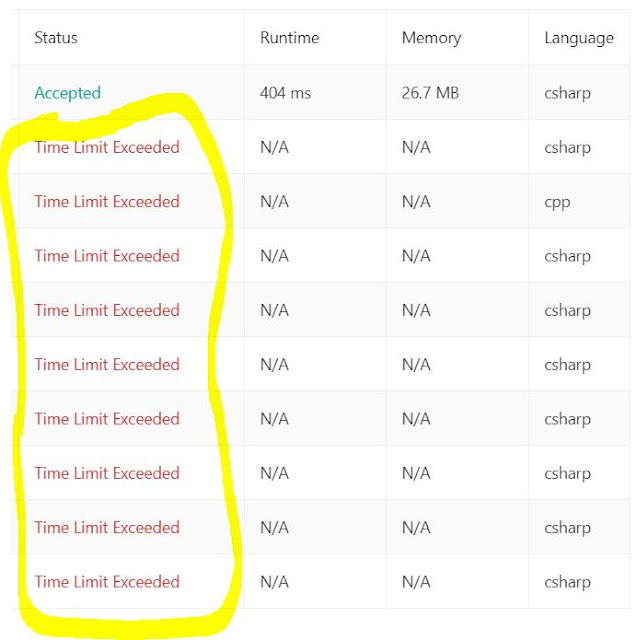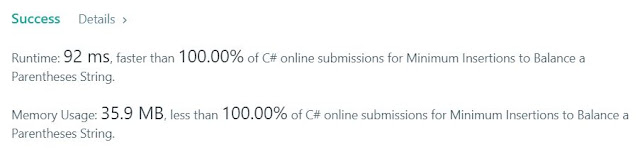## Posts

Showing posts from August, 2020

### 700The 700th LC problem that I solved reminds me of the Collatz Conjecture . Here it is  https://leetcode.com/problems/integer-replacement/ 397. Integer Replacement Medium 375 326 Add to List Share Given a positive integer  n  and you can do operations as follow: If  n  is even, replace  n  with  n /2 . If  n  is odd, you can replace  n  with either  n  + 1  or  n  - 1 . What is the minimum number of replacements needed for  n  to become 1? Example 1: Input: 8 Output: 3 Explanation: 8 -> 4 -> 2 -> 1 Example 2: Input: 7 Output: 4 Explanation: 7 -> 8 -> 4 -> 2 -> 1 or 7 -> 6 -> 3 -> 2 -> 1 The idea is to implement the rules mentioned in the problem statement, but with the following observations and optimizations:  1) Use a static hash table to keep track of the results. That's your cache 2) Use long instead of int to avoid overflows 3) Last but not least, optimize for when N is a power of 2. If N is a power of 2, the solution if Log(N,2). To ch

### Longest Repeating Substring: Sliding Window + InductionIt took me a long time to figure this one out... partially because the hints were a little misleading IMO. Here it is the problem:  https://leetcode.com/problems/longest-repeating-substring/ 1062. Longest Repeating Substring Medium 276 15 Add to List Share Given a string  S , find out the length of the longest repeating substring(s). Return  0  if no repeating substring exists.   Example 1: Input: "abcd" Output: 0 Explanation: There is no repeating substring. Example 2: Input: "abbaba" Output: 2 Explanation: The longest repeating substrings are "ab" and "ba", each of which occurs twice. Example 3: Input: "aabcaabdaab" Output: 3 Explanation: The longest repeating substring is "aab", which occurs 3 times. Example 4: Input: "aaaaa" Output: 4 Explanation: The longest repeating substring is "aaaa", which occurs twice.   Constraints: The string  S  consists of only lowercase English letters from  'a&

### Friedman NumbersI got the idea from this post from a Fermat's Library tweet: You can find more about Friedman numbers here:  https://en.wikipedia.org/wiki/Friedman_number . The "joke" in the tweet was that I had a proof about the fact that there are infinite such numbers - and the reality is that there are! But I don't really have the proof :) The code though to generate some Friedman numbers is below. I say some because I'm not taking into account parenthesis, so the set below is a subset of the Friedman numbers. It is computational intense since I need to create all the permutations of the number and then check all the possible variations with all the operators. but it finds nice ones, like this: 8^5+7*2+3 = 32785 Code is down below, cheers, ACC. *Notice that I'm using info.lundin.math library, you'll need to Nuget it first public bool IsFriedmanNumber(int number) { return IsFriedmanNumber(number.ToString(), "", new Hashtable()); } private bool IsFried

### Balancing parenthesis: use a counter, no need for a stackProblems related to balancing parenthesis usually require the use of a stack, except when the problem asks for just the counter (number of movements to balance it) or when the string consists of only one type of parenthesis, say the curly brackets. Here is an example:  https://leetcode.com/problems/minimum-insertions-to-balance-a-parentheses-string/ 1541. Minimum Insertions to Balance a Parentheses String Medium 31 8 Add to List Share Given a parentheses string  s  containing only the characters  '('  and  ')' . A parentheses string is  balanced  if: Any left parenthesis  '('  must have a corresponding two consecutive right parenthesis  '))' . Left parenthesis  '('  must go before the corresponding two consecutive right parenthesis  '))' . For example,  "())" ,  "())(())))"  and  "(())())))"  are balanced,  ")()" ,  "()))"  and  "(()))"  are not balanced. You can insert the chara